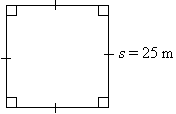# Java Program Calculate Perimeter Of Square | Programs

Java Program to calculate the perimeter of a square – In this particular piece, we will explain the various ways to calculate the perimeter of a square in Java Programming.

Suitable examples and sample programs are added for the better understanding of the given topic. The compiler has been added as well so that you can execute it yourself fairly easily.

• Using Scanner Class
• Using Command Line Arguments
• Using Static Function

As we all know, a square is a two-dimensional quadrilateral figure. It is significantly used in our daily lives.

The sides of a square are all equal in nature, along with the opposite sides being parallel to each other. The angles of a square are all equal to 90 degrees.As you can see, this is square of a side length of 25m. Thus, the perimeter of a square can be calculated as:

P = a + a + a + a = 4a.

So, the perimeter of this square is as follows:

P = 4a = 4 * 25 = 100m.

Thus, the multiple methods used to calculate the perimeter of a square in Java programming are as follows:

Output:

Output:

Output:
x

## Java Program to Add Two Matrices – 4 Ways | Programs

Java program to add two matrices – The following Java Code will let you know ...